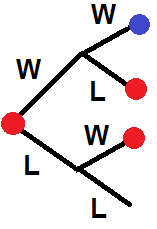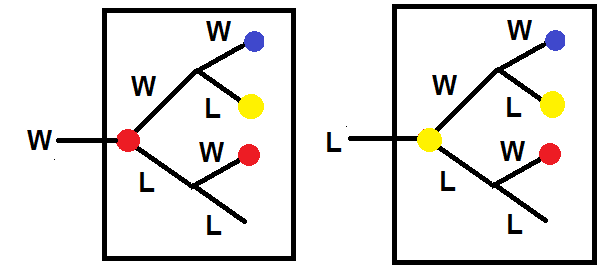You may also likeScale Invariance

By exploring the concept of scale invariance, find the probability that a random piece of real data begins with a 1.The Random World

Think that a coin toss is 50-50 heads or tails? Read on to appreciate the ever-changing and random nature of the world in which we live.Which Spinners?

Can you work out which spinners were used to generate the frequency charts?

Any Win for Tennis?

Age 16 to 18 Challenge Level:

Steve writes

Once I had understood the scoring systems I realised that I needed to look at the chances of winning based on whether I reached deuce or not. Let $V$ be the event of victory in the the overall game and $D$ the event of reaching deuce ($3$ points all) and $P_0, P_1$ and$P_2$ the probability of winning the game whilst losing $0$, $1$ and $2$ points respectively.

$$P(V) = P_0+P_1+P_2+ P(V|D)P(D)$$

$P_0, P_1$ and$P_2$ correspond to games of $4$, $5$ and $6$ points respectively. Each of these must end in a winning point (denoted W). The number of such games equals the number of ways of distributing $0$, $1$ and $2$ lost points (denoted L) amongst $3$, $4$ and $5$ points. The game reaches deuce if $6$ points are played with $3$ $W$s and $3$ $L$s. The number of such games equals the number of ways of distributing $3$ $L$s into $6$ points. Since each point is won or lost independently of the other points the probabilities multiply. Therefore,

$$P(V) = (0.6)^4\cdot 1 + (0.6)^4(0.4)\cdot 4 + (0.6)^4(0.4)^2\cdot \frac{5\cdot 4}{2}+P(V|D)\cdot(0.6)^3(0.4)^3\cdot\frac{6\cdot 5\cdot 4}{3\cdot 2}$$

I now need to use conditional probability to work out the chance of winning given that I reach deuceThe red dots on this tree indicate branches where the probability of winning equals the probability of winning given that I am at deuce $P(V|D)$ and the blue dot is a victory $V$.

$$P(V|D) = (0.6)^2 + (0.6)(0.4)P(V|D)+(0.4)(0.6)P(V|D)$$

which can be rearranged to

$$P(V|D) = \frac{(0.6)^2}{1-2(0.6)(0.4)}$$

Putting all of this together yields a probability of victory of

$$P(V) =(0.6)^4\left[ 1+ 4(0.4)+10(0.4)^2\right] +\frac{20(0.6)^5(0.4)^3}{1-2(0.6)(0.4)}$$

This equals $0.736$ (3dp). I left the probabilities in so that I could check the calculation by replacing the $0.6$ and $0.4$ by $0.5$. In this case, I get $0.5$, which makes sense (even players have an even chance of winning in this set of game rules). I also get a guaranteed victory by changing the $0.6$ to $1$ and setting the $0.4$ to $0$, which also makes sense (you will win if you win every point!)

--------------

For the second part of the problem I used a spreadsheet. It was inefficient, but displayed the probabilities clearly. After a bit of thought it is clear that the $P(V|D)$ depends on whether deuce was reached on a $W$ or on a $L$. I'll need two new events $D_W$ and $D_L$ for these cases.

We now have

$$P(V)= P(V|\bar{D})P(\bar{D})+P(V|D_L)P(D_L)+P(V|D_W)P(D_W)$$There is a fun pair of linked trees for the $P(V|D)$ - in the diagram the red circles represent $P(V|D_W)$ and the yellow circles represent $P(V|D_L)$. Reading off the probabilities gives a pair of simultaneous equations:

$$P(V|D_W) = (0.8)^2+(0.8)(0.2)P(V|D_L)+(0.2)(0.4)P(V|D_W)$$

$$P(V|D_L)=(0.4)(0.8)+(0.4)(0.2)P(V|D_L)+(0.6)(0.4)P(V|D_W)$$

This gives $P(V|D_W) = 0.792$ (exact value $\frac{80}{101}$) and $P(V|D_L) = 0.554$ (exact value $\frac{56}{101}$)

Using this piece of conditional probability to simplify the numerical analysis I then used a spreadsheet (Tennis.xls ) to calculate that

$$P(D_L) = 0.0755, \quad P(D_W) = 0.0947$$

Using this I calculate the chance of victory as

$$P(V) = 0.7364$$

For Bryony the numbers are

$$\begin{eqnarray}P(V|D_W) = 0.753\\P_(V|D_L)= 0.423\\P(D_L) = 0.0857\\P(D_W)= 0.0646\\P(V) =0.7383\end{eqnarray}$$

So, the players are very evenly matched.

You can check the results on this spreadsheet: Tennis.xls